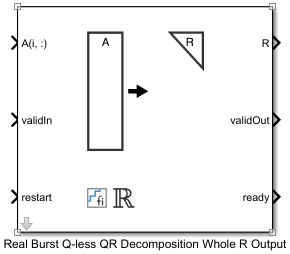# Real Burst Q-less QR Decomposition Whole R Output

Q-less QR decomposition for real-valued matrices

Since R2022b

•Libraries:
Fixed-Point Designer HDL Support / Matrices and Linear Algebra / Matrix Factorizations

## Description

The Real Burst Q-less QR Decomposition Whole R Output block uses QR decomposition to compute the economy size upper-triangular R factor of the QR decomposition A = QR, where A is a real-valued matrix, without computing Q. The solution to A'Ax = B is x = R\R'\b.

When Regularization parameter is nonzero, the Real Burst Q-less QR Decomposition Whole R Output block computes the upper-triangular factor R of the economy size QR decomposition of $\left[\begin{array}{c}\lambda {I}_{n}\\ A\end{array}\right]$ where λ is the regularization parameter.

## Ports

### Input

expand all

Rows of real matrix A, specified as a vector. A is an m-by-n matrix where m ≥ 2 and n ≥ 2. If A is a fixed-point data type, A must be signed and use binary-point scaling. Slope-bias representation is not supported for fixed-point data types.

Data Types: `single` | `double` | `fixed point`

Whether inputs are valid, specified as a Boolean scalar. This control signal indicates when the data from the `A(i,:)` input port is valid. When this value is 1 (`true`) and the value of `ready` is 1 (`true`), the block captures the values at the `A(i,:)` input port. When this value is 0 (`false`), the block ignores the input samples.

After sending a `true` `validIn` signal, there may be some delay before `ready` is set to `false`. To ensure all data is processed, you must wait until `ready` is set to `false` before sending another `true` `validIn` signal.

Data Types: `Boolean`

Whether to clear internal states, specified as a Boolean scalar. When this value is 1 (`true`), the block stops the current calculation and clears all internal states. When this value is 0 (`false`) and the value at `validIn` is 1 (`true`), the block begins a new subframe.

Data Types: `Boolean`

### Output

expand all

Economy size QR decomposition matrix R, returned as a vector. R is an upper triangular matrix. The size of matrix R is `min(m,n)`-by-`n`. R has the same data type as A.

Data Types: `single` | `double` | `fixed point`

Whether the output data is valid, specified as a Boolean scalar. This control signal indicates when the data at output port `R(i,:)` is valid. When this value is 1 (`true`), the block has successfully computed the matrix R. When this value is 0 (`false`), the output data is not valid.

Data Types: `Boolean`

Whether the block is ready, returned as a Boolean scalar. This control signal indicates when the block is ready for new input data. When this value is `1` (`true`) and `validIn` is `1` (`true`), the block accepts input data in the next time step. When this value is `0` (`false`), the block ignores input data in the next time step.

After sending a `true` `validIn` signal, there may be some delay before `ready` is set to `false`. To ensure all data is processed, you must wait until `ready` is set to `false` before sending another `true` `validIn` signal.

Data Types: `Boolean`

## Parameters

expand all

Number of rows in input matrix A, specified as a positive integer-valued scalar.

#### Programmatic Use

 Block Parameter: `m` Type: character vector Values: positive integer-valued scalar Default: `4`

Number of columns in input matrix A, specified as a positive integer-valued scalar.

#### Programmatic Use

 Block Parameter: `n` Type: character vector Values: positive integer-valued scalar Default: `4`

Regularization parameter, specified as a real nonnegative scalar. Small, positive values of the regularization parameter can improve the conditioning of the problem and reduce the variance of the estimates. While biased, the reduced variance of the estimate often results in a smaller mean squared error when compared to least-squares estimates.

#### Programmatic Use

 Block Parameter: `regularizationParameter` Type: character vector Values: real nonnegative scalar Default: `0`

expand all